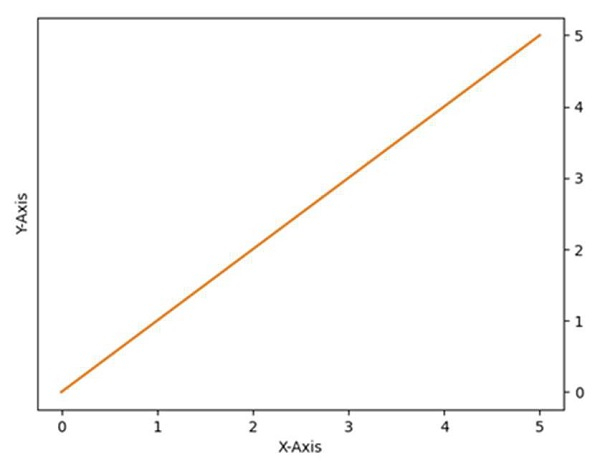# How to move the Y-axis ticks from the left side of the plot to the right side in matplotlib?

MatplotlibServer Side ProgrammingProgrammingMachine Learning

To shift the Y-axis ticks from left to right, we can perform the following steps −

• Create a figure using the figure() method.

• Using the above figure method, create the axis of the plot, using add_subplot(xyz), where x is row, y is column, and z is index.

• To shift the Y-axis ticks from left to right, use ax.yaxis.tick_right() where ax is axis created using add_subplot(xyz) method.

• Now plot the line using plot() method, with given x and y points, where x and y points can be created using np.array() method.

• Set up x and y labels, e.g., X-axis and Y-axis , using xlabel and ylabel methods.

• Use plt.show() to show the figure.

## Example

from matplotlib import pyplot as plt
import numpy as np

f = plt.figure()
ax.yaxis.tick_right()
xpoints = np.array([0, 5])
ypoints = np.array([0, 5])
plt.plot(xpoints, ypoints)

plt.ylabel("Y-axis ")
plt.xlabel("X-axis ")

plt.show()

## Output